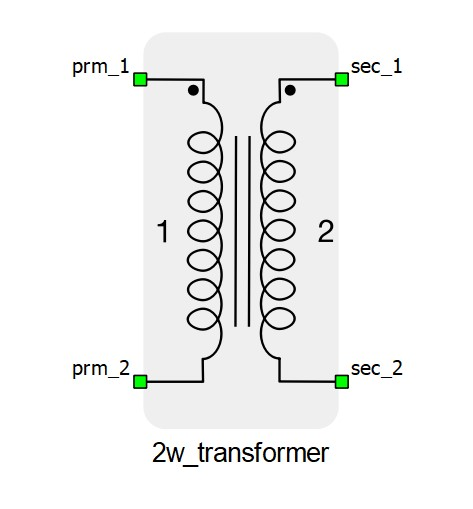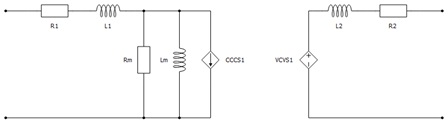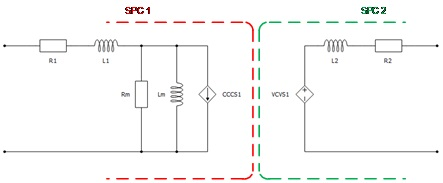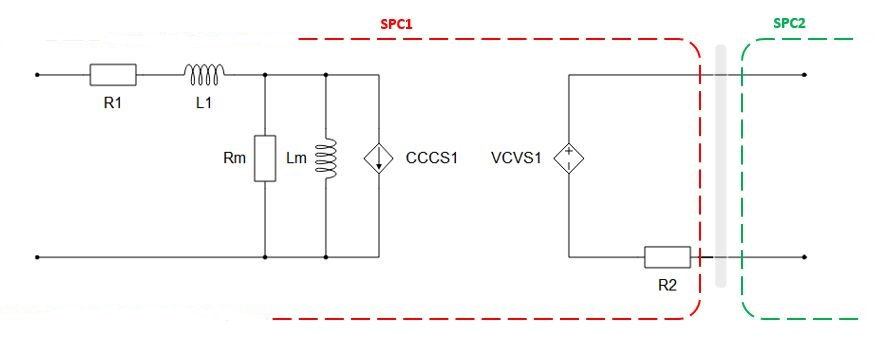# Single phase two winding transformer

This section describes single phase two winding transformer

The single-phase two-winding transformer models two coupled windings on the same core. The magnetization inductance Lm can be linear or with saturation, it is modeled on the primary side of the transformer. Core losses are modeled as Rm resistance located on the primary side of the transformer. Also, it is possible to neglect Lm and Rm by selecting Lm/Rm neglected in the Core model property. For more informations, please refer to Core model

The schematic symbol and input parameters for the single-phase two-winding transformer block are given in Table 1.

Table 1. The single-phase two-winding transformer component in the HIL Toolbox
component component dialog window component parametersSingle phase two winding transformer• Input parameters option – SI/p.u.
• Nominal Power of the transformer in VA (Sn)
• Nominal frequency in Hz (fn)
• Nominal voltage of the primary winding (n1)
• Nominal voltage of the secondary winding (n2)
• Leakage inductance of the primary winding (L1)
• Initial current on primary side (I1)
• Leakage inductance of the secondary winding (L2)
• Initial current on secondary side (I2)
• Core model - Linear, Non-Linear, Rm/Lm neglected
• Magnetization Inductance (Lm)
• Primary winding resistance (R1)
• Secondary winding resistance (R2)
• Equivalent resistance representing the iron core losses (Rm)
• Import from PU button• Embedded coupling - inserts a coupling component (Ideal Transformer based or TLM) in transformer.
• Type of coupling component - defines whether the inserted coupling component is a core or a device coupling. Device coupling is available only for the Ideal Transformer based coupling.
• TLM/Embedded components ratio - Defines how coupling to embedded inductors ratio will be calculated. Available only for the TLM coupling.
• Ratio - Used to specify coupling to embedded inductors ratio if Manual option is selected in TLM/Embedded components ratio property.

A schematic block diagram of the single phase two winding transformer block with the corresponding component arrangement and naming is shown in Figure 1.Figure 1. The schematic block diagram of a single-phase two-winding transformer with corresponding components naming

## Embedded coupling

There are two possible options for embedded coupling in the single phase two winding transformer, Ideal Transformer based coupling and TLM coupling.

If Embedded coupling is set to Ideal Transformer, Ideal Transformer based coupling will be placed between two windings of transformer. In Figure 2 is given a schematic representation of transformer's equivalent circuit when Embedded coupling is set to Ideal Transformer.Figure 2. The schematic block diagram of a single-phase two-winding transformer with inserted Ideal Transformer based coupling component

If Embedded coupling is set to TLM, secondary winding inductor (L2) will be replaced with TLM coupling component. Inductance will be divided between coupling and embedded inductors (inductors will be hidden in the TLM). TLM to embedded inductors ratio can be determined by compiler, but also it can be specified explicitly. If Automatic option is selected, ratio will be determined by discretization method. If Manual option is selected, ratio can be explicitly set to meet user requirements. For more information on TLM couplings please refer to Core couplings - TLM. In Figure 3 is given a schematic representation of transformer's equivalent circuit when Embedded coupling is set to TLM.Figure 3. The schematic block diagram of a single-phase two-winding transformer with inserted TLM coupling component

## State variable naming for the single-phase two-winding transformer (internal to the component)

Analog output variable name Description
(name).L1 Primary winding current
(name).L2 Second winding current
(name).Lm Magnetizing current